The traditional approach to determining salient loadings (loadings that are considered large in absolute values) employs rules of thumb such as 0.3 or 0.4. However, this does not use the statistical evidence efficiently. The asymptotic normality of the distribution of factor loadings enables you to construct confidence intervals to gauge the salience of factor loadings. To guarantee the range-respecting properties of confidence intervals, a transformation procedure such as in CEFA (Browne et al.; 2008) is used. For example, because the orthogonal rotated factor loading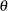must be bounded between1 and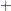1, the Fisher transformation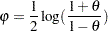is employed so that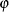is an unbounded parameter. Assuming the asymptotic normality of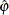, a symmetric confidence interval foris constructed. Then, a back-transformation on the confidence limits yields an asymmetric confidence interval for. Applying the results of Browne (1982), a (1
)100% confidence interval for the orthogonal factor loadingis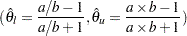where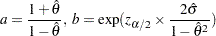and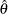is the estimated factor loading,
is the standard error estimate of the factor loading, and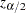is the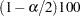percentile point of a standard normal distribution.

Once the confidence limits are constructed, you can use the corresponding coverage displays for determining the salience of the variable-factor relationship. In a coverage display, the COVER= value is represented by an asterisk (*). The following table summarizes various displays and their interpretations.

Table 34.2 Interpretations of the Coverage Displays

Positive Estimate

Negative Estimate

COVER=0 Specified

Interpretation

*

*

The estimate is not significantly different from zero, and the CI covers a region of values that are smaller in magnitude than the COVERvalue. This is strong statistical evidence for the nonsalience of the variable-factor relationship.

0[ ]*

*[ ]0

The estimate is significantly different from zero, but the CI covers a region of values that are smaller in magnitude than the COVERvalue. This is strong statistical evidence for the nonsalience of the variable-factor relationship.

[0*]

[*0]



The estimate is not significantly different from zero or the COVERvalue. The population value might have been larger or smaller in magnitude than the COVERvalue. There is no statistical evidence for the salience of the variable-factor relationship.

0[*]

[*]0

The estimate is significantly different from zero but not from the COVERvalue. This is marginal statistical evidence for the salience of the variable-factor relationship.

0*[ ]

[ ]*0

0[ ] or [ ]0

The estimate is significantly different from zero, and the CI covers a region of values that are larger in magnitude than the COVERvalue. This is strong statistical evidence for the salience of the variable-factor relationship.

See Example 34.4 for an illustration of the use of confidence intervals for interpreting factors.